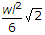# Civil Engineering - UPSC Civil Service Exam Questions

16.

Undrained strength of a clay soil can be obtained by conducting which of the following tests ?
1. UC test
2. Vane sheer test
3. Cyclic triaxial test
4. Slow direct sheer test
Select the correct answer using the code given below :

 A. 1 and 2 only B. 2 only C. 1 and 4 D. 1, 2 and 3

Explanation:

No answer description available for this question. Let us discuss.

17.

Which one of the following is the nominal size of standard modular brick ?

 A. 25cm x 13cm x 8cm B. 25cm x 10cm x 8cm C. 20cm x 10cm x 10cm D. 20cm x 15cm x 10cm

Explanation:

No answer description available for this question. Let us discuss.

18.

What is the ratio of rate of back-washing to that of filtration in a typical rapid sand filter ?

 A. 2 B. 4 C. 6 D. 10

Explanation:

No answer description available for this question. Let us discuss.

19.

A point in an element is stressed as shown in the given figure. What is the value of normal stress on the oblique plane BE ?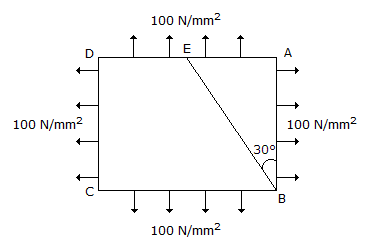A. 200 N / mm2 B. 175 N / mm2 C. 150 N / mm2 D. 100 N / mm2

Explanation:

No answer description available for this question. Let us discuss.

20.

What is the maximum bending moment at mid-span of the beam given below ?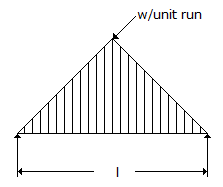A.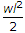B.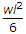C.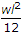D.